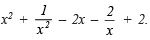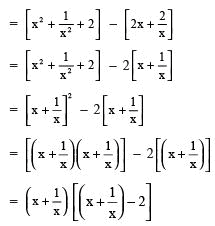Practice Questions: Polynomials

# Practice Questions: Polynomials | Mathematics (Maths) Class 9

 1 Crore+ students have signed up on EduRev. Have you?

Question 1. Show that (x + 3) is a factor of x3 + x2 – 4x + 6.
Solution:
∵ p(x) = x+ x2 – 4x + 6
Since (x + 3) is a factor, then x + 3 = 0 ⇒ x = – 3
∴ p( – 3) = ( – 3)3 + ( – 3) – 4( – 3) + 6
= – 27 + 9 + 12 + 6
= 0
∴ (x + 3) is a factor of x+ x2 – 4x + 6

Question 2. Show that (x – 5) is a factor of: x3 – 3x – 13x + 15
Solution:
∵ p(x) = x3 – 3x2 – 13x + 15
Since (x – 5) is a factor, then x – 5 = 0
⇒ x = 5
∴ p(5) = (5) – 3(5) – 13(5) + 15
= 125 – 75 – 65 + 15 = 140 – 140 = 0
∴ (x – 5) is a factor of x – 3x2 – 13x + 15.

Question 3. Find the value of a such that (x + α ) is a factor of the polynomial
f(x) = x4 – α 2x2 + 2x + α + 3.
Solution:
Here f(x) = xα2 x2 + 2x + α + 3
Since, (x + a) is a factor of f(x)
∴ f ( – α) = 0
⇒ ( – α)4α2 ( – α)2 + 2( – α) + a + 3 = 0
α4α4 – 2α + α + 3= 0
⇒ – α + 3 = 0
α = 3

Question 4. Show that (x – 2) is a factor of 3x3 + x2 – 20x + 12.
Solution:
f(x) = 3x3 + x2 – 20x + 12
For (x – 2) being a factor of f(x), then x – 2 = 0
⇒ x = 2
∴ f(2) must be zero.
Since f(2) = 3(2)3 + (2)2 – 20(2) + 12
= 3(8) + 4 – 40 + 12
= 24 + 4 – 40 + 12
= 40 – 40 = 0
which proves that (x – 2) is a factor of f(x).

Question 5. Factorise the polynomialSolution: We haveQuestion 6. Factorise a(a – 1) – b(b – 1).
Solution:
We have a(a – 1) – b(b – 1)
= a2 – a – b2 + b
= a2 – b2 – (a – b)
(Rearranging the terms) = [(a + b)(a – b)] – (a – b)
[∵ x– y2 = (x + y)(x – y)]
= (a – b)[(a + b) – 1]
= (a – b) (a + b – 1)
Thus, a(a – b) – b(b – 1) = (a – b)(a + b – 1)

Question 7. Show that x3 + y3 = (x + y )(x2 – xy + y2).
Solution:
Since (x + y)3 = x3 + y+ 3xy(x + y)
∴ x3 + y3 = [(x + y)3] –3xy(x + y)
= [(x + y)(x + y)2] – 3xy(x + y)
= (x + y)[(x + y)2 – 3xy]
= (x + y)[(x+ y2 + 2xy) – 3xy]
= (x + y)[x2 + y2 – xy]
= (x + y)[x2 + y2 – xy]
Thus, x3 + y3 = (x + y)(x2 – xy + y2)

Question 8. Show that x3 – y3 = (x – y)(x+ xy + y2).
Solution
: Since (x – y)3 = x3 – y3 – 3xy(x – y)
∴   x3 – y3 = (x – y)3 + 3xy(x – y)
= [(x – y)(x – y)2] + 3xy(x – y)
= (x – y)[(x – y)2 + 3xy]
= (x – y)[(x2 + y2 – 2xy) + 3xy]
= (x – y)[x2 + y2 – 2xy + 3xy]
= (x – y)(x2 + y2 + xy)
Thus, x3 – y3 = (x – y)(x2 + xy + y2)

The document Practice Questions: Polynomials | Mathematics (Maths) Class 9 is a part of the Class 9 Course Mathematics (Maths) Class 9.
All you need of Class 9 at this link: Class 9

## Mathematics (Maths) Class 9

76 videos|397 docs|109 tests

## Mathematics (Maths) Class 9

76 videos|397 docs|109 tests

Track your progress, build streaks, highlight & save important lessons and more!(Scan QR code)

,

,

,

,

,

,

,

,

,

,

,

,

,

,

,

,

,

,

,

,

,

;